# Parallel sailing

By parallel sailing we mean travelling along a parallel of latitude from one place to another. This means that one would either be travelling directly westward or eastward [270° (T) or 090° (T)]. The distance along a parallel of latitude between two particular meridians decreases as the meridians converge towards the poles. The distance decreases as the latitude increases. Because of this, the distance along a chosen latitude is referred to as “Departure” and it is expressed in nautical miles.
To determine the distance or departure between two positions, the following formula is used: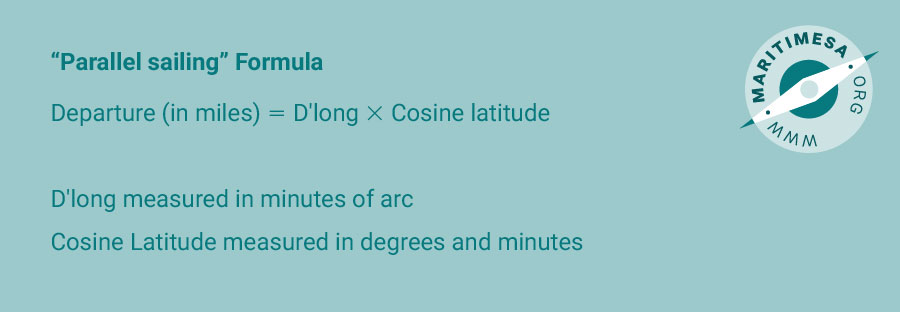See Appendix A below for the derivation of this formula.

For example: The latitude of the parallel is 40° S and the longitudes of the two positions are 15° E and 60°E respectively. Subtracting the one longitude from the other we get a d’long of 45° or 2 700’. To calculate the distance along the parallel (departure) we apply the above data to the formula as follows: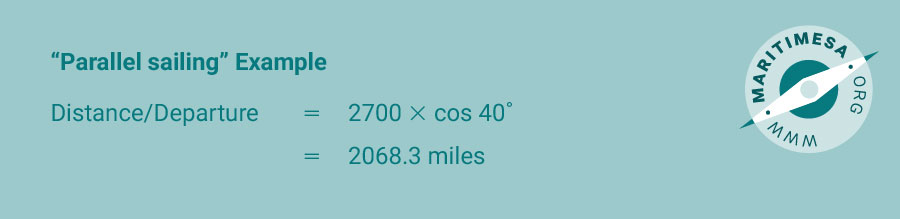In help memorize the formula use the following figure: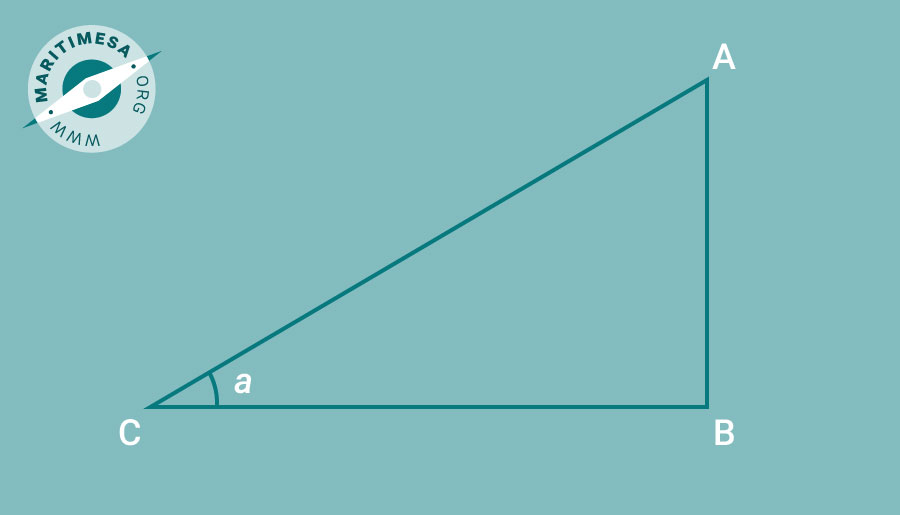Side CA is the d’long, side CB is the departure and the angle ACB is the latitude.

As a guide, a pro-forma of the method used is attached below as Appendix B entitled ‘Parallel Sailing’.

Appendix A.
Derivation of the parallel sailing formula: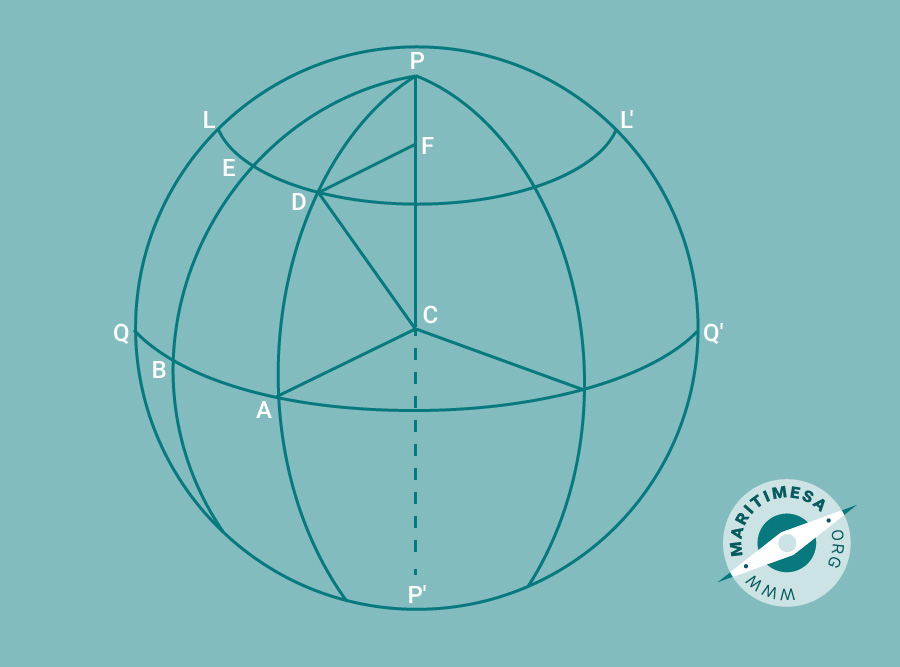Derivation of the parallel sailing formula.

The above figure represents the earth where P, P’ represents the two poles, Q, Q’ represents the equator , L, L represents a parallel latitude, C represents the middle of the earth and lastly F that represents the centre of the small circle L, L’.

E and D are two positions on latitude L, L’. PAP’ and PBP’ are meridians passing through positions E and D while CA, CB and CD are radii of the earth. According to circular measure, the length of an arc, which subtends any given angle at its centre, is proportional to the radius. From this, DE/DF = BA/AC, where DE is the departure and BA is the d.long. Therefore DE/BA = DF/AC and since DC and AC are both radii of the earth, DE/BA = DF/DC. It follows therefore that DE/BA = cosine angle FDC.
Thus departure/d.long = cosine latitude.

Appendix B.
Pro-forma for parallel sailing.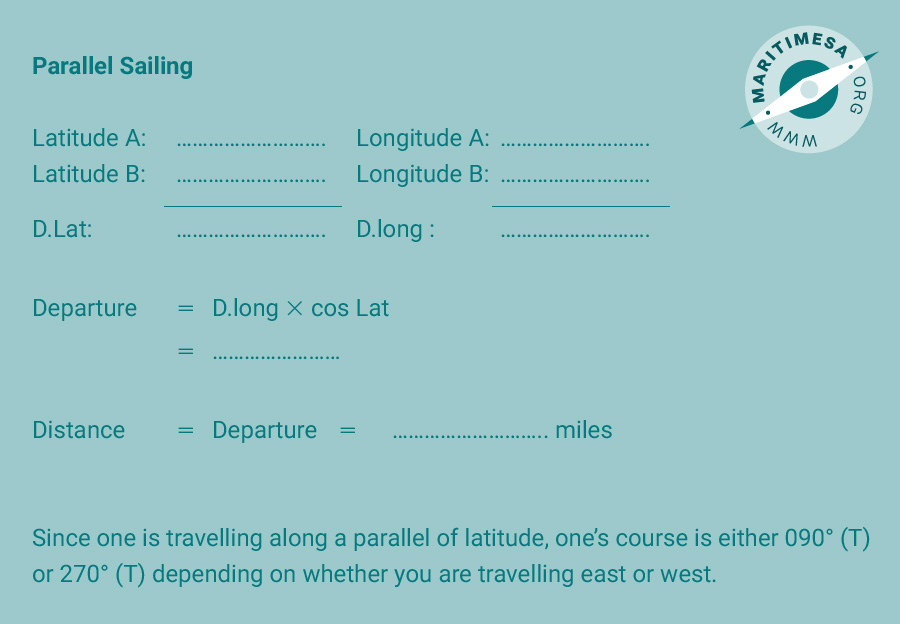Pro-forma for parallel sailing.